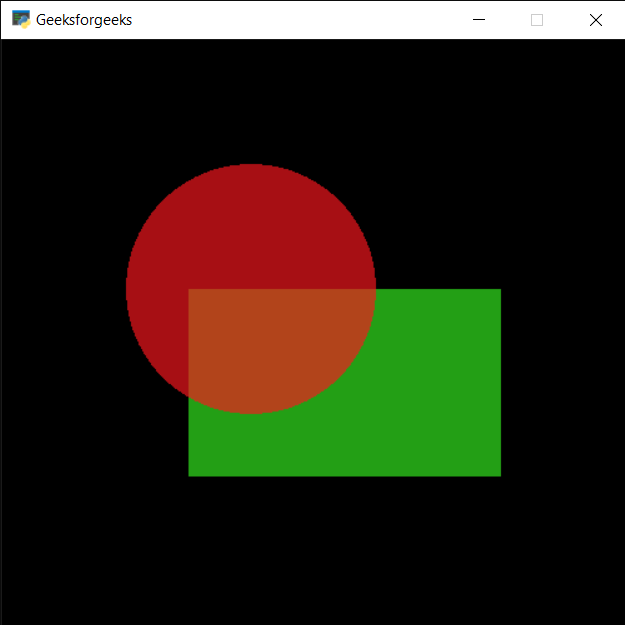Related Articles

# PYGLET – Shape Width

• Last Updated : 09 Jul, 2021

In this article we will see how we can access the width of the shape in PYGLET module in python. Pyglet is easy to use but powerful library for developing visually rich GUI applications like games, multimedia etc. A window is a “heavyweight” object occupying operating system resources. Windows may appear as floating regions or can be set to fill an entire screen (fullscreen). A shape is the form of an object or its external boundary, outline, or external surface, as opposed to other properties such as color, texture or material type. Many shapes are drawn with the help of shapes module of pyglet. Width is defined as the quality of being wide, or the measurement of distance from side to side. For shapes like circle there is radius in place of width
We can create a window with the help of command given below

```# creating a window
window = pyglet.window.Window(width, height, title)```

Attention geek! Strengthen your foundations with the Python Programming Foundation Course and learn the basics.

To begin with, your interview preparations Enhance your Data Structures concepts with the Python DS Course. And to begin with your Machine Learning Journey, join the Machine Learning - Basic Level Course

In order to create window we use width attribute with the shape
Syntax : shape.width
Argument : It takes no argument
Return : It returns integer

Below is the implementation

## Python3

 `# importing pyglet module``import` `pyglet` `# importing shapes from the pyglet``from` `pyglet ``import` `shapes` `# width of window``width ``=` `500``  ` `# height of window``height ``=` `500``  ` `# caption i.e title of the window``title ``=` `"Geeksforgeeks"``  ` `# creating a window``window ``=` `pyglet.window.Window(width, height, title)` `# creating a batch object``batch ``=` `pyglet.graphics.Batch()`  `# properties of rectangle``# co-ordinates of rectangle``co_x ``=` `150``co_y ``=` `150` `# width of rectangle``width ``=` `250` `# height of rectangle``height ``=` `150` `# color = green``color ``=` `(``50``, ``225``, ``30``)` `# creating a rectangle``rec ``=` `shapes.Rectangle(co_x, co_y, width, height, color ``=` `color, batch ``=` `batch)` `# changing opacity of the rect1``# opacity is visibility (0 = invisible, 255 means visible)``rec.opacity ``=` `180`  `# creating another rectangle with properties``# x, y co ordinate : 50, 250``# width, height of rectangle : 300, 200``# color = red``color ``=` `(``255``, ``25``, ``25``)` `# properties of circle``# co-ordinates of circle``circle_x ``=` `200``circle_y ``=` `300` `# size of circle``# color = green``size_circle ``=` `100` `# creating a circle``circle ``=` `shapes.Circle(circle_x, circle_y, size_circle, color ``=``(``250``, ``22``, ``30``), batch ``=` `batch)` `# changing opacity of the circle1``# opacity is visibility (0 = invisible, 255 means visible)``circle.opacity ``=` `170`  `# window draw event``@window``.event``def` `on_draw():``    ` `    ``# clear the window``    ``window.clear()``    ` `    ``# draw the batch``    ``batch.draw()``    ` `    `  `# accessing width of rectangle``value_rec ``=` `rec.width` `# printing value``print``(``"Rectangle : "``, end ``=` `"")``print``(value_rec)` `# accessing radius of circle``value_cir ``=` `circle.radius` `# printing value``print``(``"Circle : "``, end ``=` `"")``print``(value_cir)`   `# run the pyglet application``pyglet.app.run()`

Output :```Rectangle : 250
Circle : 100```

My Personal Notes arrow_drop_up